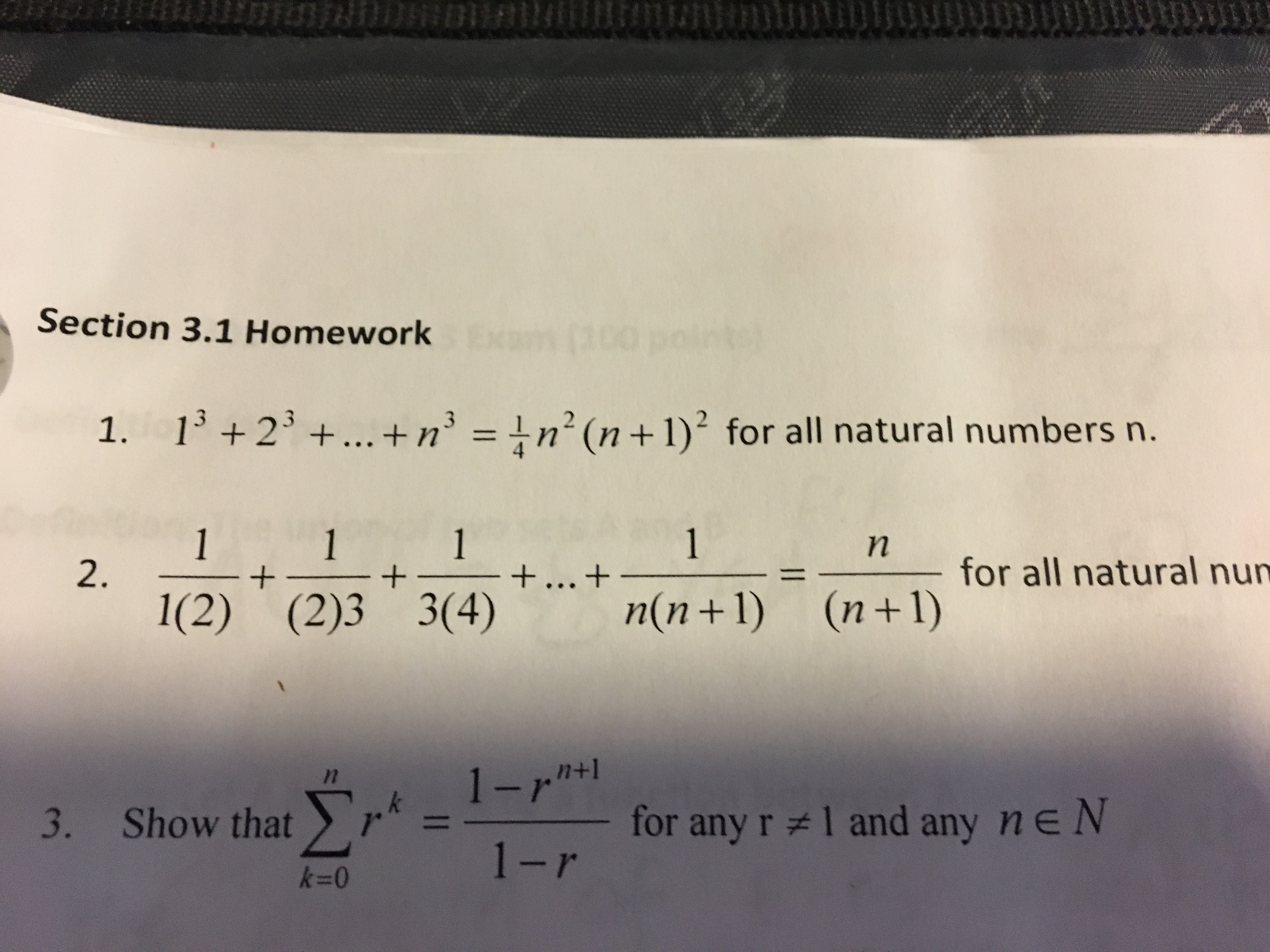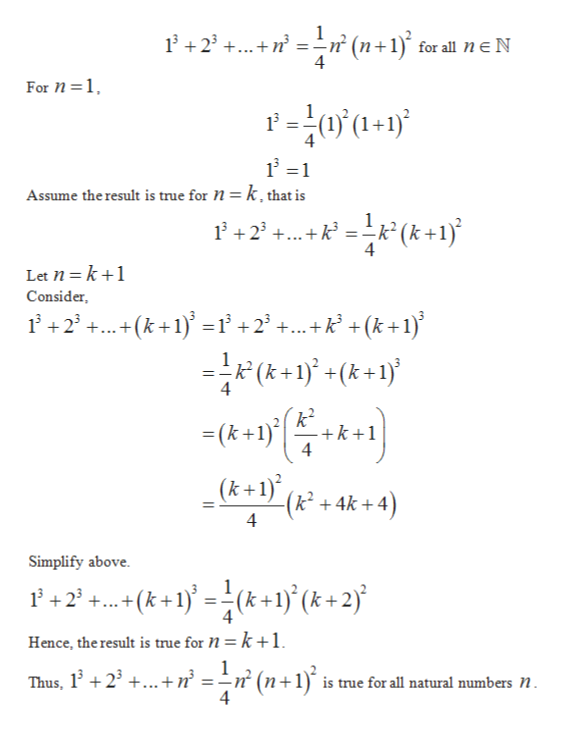# WeSection 3.1 Homework1.1+2+... +n' = 1n2 (n +1) for all natural numbers n.1+... +n(n + 1)11+1n2.+for all natural num1(2) (2)3 3(4)(n 1)(n+1)n+1- for any r 1 and any ne N1-r3. Show that

Question
2 views

#1help_outlineImage TranscriptioncloseWe Section 3.1 Homework 1. 1+2+... +n' = 1n2 (n +1) for all natural numbers n. 1 +... + n(n + 1) 1 1 + 1 n 2. + for all natural num 1(2) (2)3 3(4) (n 1) (n+1) n+1 - for any r 1 and any ne N 1-r 3. Show that fullscreen
check_circle

star
star
star
star
star
1 Rating
Step 1

We prove this by mathematical in...help_outlineImage Transcriptionclose1+2+nn? (n+1) for all n E N For n 1 Assume the result is true for N = k, that is P+2t k+ 1) Let n k1 Consider +2(k+1)1+2 +... + k? + (k+1) 1 (k 4 -k+1 4 (k +1\ (k + 4k + 4) 4 Simplify above (k+1}={(k+1}(& + 2j +2 4 Hence, the result is true for = k +1 Thus, 123...+ n = -n (n+1 is true for all natural numbers N. 4 fullscreen

### Want to see the full answer?

See Solution

#### Want to see this answer and more?

Solutions are written by subject experts who are available 24/7. Questions are typically answered within 1 hour.*

See Solution
*Response times may vary by subject and question.
Tagged in

### Math